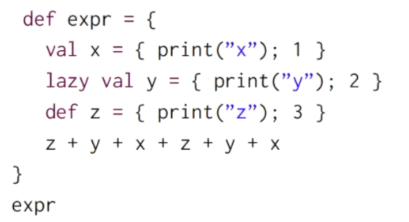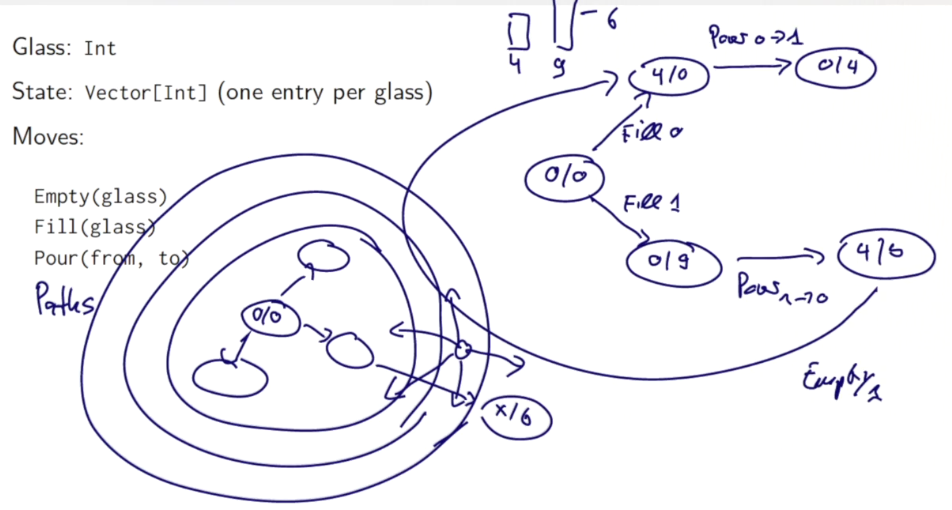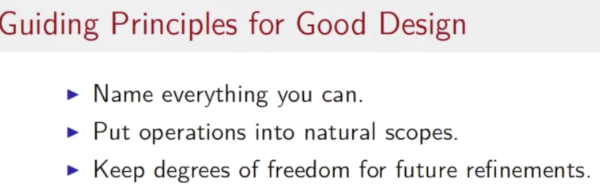# Lecture 2.1 - Structural Induction on Trees (optional)

Generalize the structural induction on list to general structures like trees.

To prove a property `P(t)` for all trees `t`:
* show for any leave l, `P(l)` holds
* for each internal node `t` with subtrees `s1...sn`, show `P(s1)&...&P(sn)` holds

## Example: Prove correctness for IntSets

recall the `IntSet` def: empty set or non-empty set organized in a binary (search) tree structure.

```abstract class IntSet{
def incl(x: Int): IntSet
def contains(x: Int): Boolean
}
object Empty extends IntSet{
def contains(x: Int): Boolean = false
def incl(x: Int): IntSet = NonEmpty(x, Empty, Empty)
}
class NonEmpty(elem: Int, left: IntSet, right: IntSet) extends IntSet{
// NonEmpty is just a BST
def contains(x: Int): Boolean =
if (x<elem) left contains x
else if (x>elem) right contains x
else true
def incl(x: Int): IntSet =
if (x<elem) NonEmpty(elem, left incl x, right)
else if (x>elem) NonEmpty(elem, left, right incl x)
else this
}
```

Prove the correctness of this implementation: show that it respects certain laws:

prop1: `Empty contains x = false`

pf: easy...

prop2: `(s incl x) contains x = true`

pf: proof by structual induction on `s`

• base case: `s = Empty`,

```(Empty incl x) = NonEmpty(x, Empty, Empty)
NonEmpty(x, Empty, Empty) contains x = true
```
• induction step: `s=NonEmpty(z,l,r)`

• first case, `z=x` s incl x = NonEmpty(x,l,r) incl x = NonEmpty(x,l,r)

so `NonEmpty(x, l, r) contains x = true` - second case, z<x `s incl x = NonEmpty(z,l,r) incl x = NonEmpty(z,l,r incl x)` so:

```(s incl x) contains x
= NonEmpty(z,l,r incl x) contains x
= (r incl x) contains x = ture // by induction hypothesis
```
• third case, z>x: symmetric ...

prop3: if x!=y, `(s incl x) contains y = s contains y`

pf: by structual induction

• base case: `s = Empty`
• induction step: `s=NonEmpty(z,l,r)` 5 cases to consider...
• z=x
• z=y
• z<y<x
• y<z<x
• y<x<z

# Lecture 2.2 - Streams

motivation: 2nd prime number between 1000 and 10000:

elegant, but not efficient solution:
`( (1000 to 10000) filter isPrime) (1)`

→ ALL prime numbers are constructed in the code, only use the first 2 elements...

⇒ *avoid computing the tail of a seq until necessary *

→ new data structure: `Stream`s, like lists, but tail is eval only on demand

## Stream class

constructor Streams defined from either constant `Stream.empty` and constructor `Stream.cons` ex: `val xs = Stream.cons(1, Stram.cons(2, Stream.empty))`

(Stream.empty~=`Nil`, Stream.cons~=`::`)

or by using the `Stream` object as a factory (like other collections): `val xs = Stream(1,2,3)`

toStream() `toStream` on a collection will turn the collection into a stream.

```    scala> (1 to 1000).toStream
res1: scala.collection.immutable.Stream[Int] = Stream(1, ?) // the tail is (?) -- not evaled
```

The tail is not evaled!

let's write a function to return a range as Stream ( returns `(lo until hi).toStream)` ):

```def streamRange(lo: Int, hi: Int): Stream[Int] =
if (lo>hi) stream.empty)
else Stream.cons(lo, stramRange(lo+1, hi))
```

other methods `Stream` supports almost all method of a `List`

`((1000 to 10000).toStream filter isPrime)(1)`

Exception: concat operator `::` will always return a list instead of a stream. → alternative: `#::` returns a stream `x#::xs == Stream.cons(x,xs)`

## Implementation of Streams

similar to the implementation of lists.

```scala trait Stream[+A] extends Seq[A]{ def isEmpty: Boolean def head: A def tail: Stream [A] }```

concrete implementations are in the `Stream` companion object:

```object Stream{
def cons[T](hd:T, tl: => Stream[T]) = // **`=>`: tl is by name, will be evaled later!
new Stream[T]{
def isEmpty = false
def tail = tl
}
val empty = new Stream[Nothing]{
def isEmpty = true
def tail = throw new NoSuchElementException("empty.tail")
}
}
```

all other methods are implemented similar to list counterparts...
ex:

```class Stream[+T]{
//...
def filter(p: T=>Boolean): Stream[T] =
if (isEmpty) this
else tail.filter(p)
//...
}
```

# Lecture 2.3 - Lazy Evaluation

pb with the last implementation of Streams: if tail is called several times, the corresponding stram will be re-computed each time.

→ can store the 1st result of the eval and use it later. (As in FP, the eval result never change)

⇒ this scheme is called lazy evaluation, as opposed to both by-name evaluation (`def x`) and strict-evaluation (`val x`). avoids both unnessary and repeated eval.

`lazy val x = expr`

exercice:→ this will print: "xzyz"

adapt the lazy scheme for Stream implementation:

```def cons[T](hd: T, tl: => Stream[T]) =
new Stream[T]{
lazy val tail = tl // **lazy instead of `def`**
//...
}
```

# Lecture 2.4 - Computing with Infinite Sequences

with lazyness, can deal with inf seqs. ⇒ infinite Streams

example: the stream of all integers starting from a given number:

```def from(n: Int): Stream[Int] =
n #:: from(n+1) // non-terminating recursive call here
```

all natural numbers:
`val nats = from(0)` all multiples of 4:
`nats map (_ * 4)`

```scala> def from(n:Int):Stream[Int] = n #:: from(n+1);
from: (n: Int)Stream[Int]

scala> val nats = from(0)
nats: Stream[Int] = Stream(0, ?)

scala> val m4s = nats map (_ * 4)
m4s: scala.collection.immutable.Stream[Int] = Stream(0, ?)

scala> (m4s take 10).toList
res2: List[Int] = List(0, 4, 8, 12, 16, 20, 24, 28, 32, 36)
```

example1: Sieve of Eratosthenes
-------------------------------

![](../images/progfun2_lec2_lazyeval/pasted_image001.png)

written in scala:

```scala
def sieve(s:Stream[Int]): Stream[Int] = // param s is the prime numbers for sieve

scala> val primes = sieve(from(2))
primes: Stream[Int] = Stream(2, ?)

scala> primes.take(100).toList
res0: List[Int] = List(2, 3, 5, 7, 11, 13, 17, 19, 23, 29, 31, 37, 41, 43, 47, 53, 59, 61, 67, 71, 73, 79, 83, 89, 97, 101, 103, 107, 109, 113, 127, 131, 137, 139, 149, 151, 157, 163, 167, 173, 179, 181, 191, 193, 197, 199, 211, 223, 227, 229, 233, 239, 241, 251, 257, 263, 269, 271, 277, 281, 283, 293, 307, 311, 313, 317, 331, 337, 347, 349, 353, 359, 367, 373, 379, 383, 389, 397, 401, 409, 419, 421, 431, 433, 439, 443, 449, 457, 461, 463, 467, 479, 487, 491, 499, 503, 509, 521, 523, 541)
```

## example2: square root

previous implementation: use `isGoodEnough` test termination. now: express the converging seq without having to worry about when to terminate:

```def sqrtStream(x: Double): Stream[Double] = {
def improve(guess: Double) = (guess + x/guess) / 2
lazy val guesses: Stream[Double] = 1 #:: (guesses map improve) //**this will not explod!**
guesses
}
def isGoodEnough(guess: Double, x: Double) =
math.abs((guess * guess - x) / x) < 0.0001
(sqrtStream(4) filter (isGoodEnough(_, 4)) ) (1) // get the 1st guess that is good enough
```

# Lecture 2.5 - Case Study: the Water Pouring Problem

task: "water pouring problem" given: source, sink, glasses of different sizes, target size...
goal: fill/empty/move water from 1 glass to anotherModel Glass: `Int` State: `Vector[Int]` one entry per glass Moves:
`Empty(glass), Fill(glass), Pour(from, to)`question: how to find the correct sequence of moves ?
⇒ BFS, generate all possible paths (from initial state where all glasses are empty) of length=1,2,3,...

Implementation

first: define Move classes, they have a change method, take one state as param and return a state:

```class Pouring(capacity:Vector[Int]) {
type State = Vector[Int]
val initialState = capacity map ( x => 0 )

trait Move{
def change(state:State):State
}
case class Empty(glass:Int) extends Move{
def change(state: State) = state.updated(glass,0)
}
case class Fill(glass:Int) extends Move{
def change(state: State) = state.updated(glass,capacity(glass))
}
case class Pour(from:Int, to:Int) extends Move{
def change(state: State) ={
val amount = state(from) min (capacity(to)-state(to)) //the amount to move
state updated (from, state(from)-amount) updated (to, state(to)+amount)
}
}
...
}
```

generate all possible moves:

```val glasses = capacity.indices // equal to `0 until capacity.length`

val moves =  // all possible moves
( for(g<-glasses) yield Empty(g) ) ++
( for(g<-glasses) yield Fill(g) ) ++
( for(gfrom<-glasses; gto<-glasses; if gfrom!=gto) yield Pour(gfrom, gto) )
```

Then define a `Path` class:

```  class Path(history: List[Move]){// history: moves in reversed order
def endState:State = // given a path (list ot moves), return the final state by following the path
(history foldRight(initialState)) ( (mv:Move, stt:State) => mv change stt) // as in the list are reversed order of moves, use foldright

def extend(move: Move) = new Path(move::history)

override def toString =
( history.reverse mkString " " ) + "-->" + endState
}

val initialPath = new Path(Nil)
```

Then define a `Stream` of path sets: given current (set of) paths, extend one move and generate (a set of) paths of longer length:

```  def from(paths: Set[Path]): Stream[Set[Path]] ={
if(paths.isEmpty) Stream.empty
else {
val more = for{
path<-paths
next <- moves map path.extend
}yield next
paths #:: from(more)
}
}
val pathSets = from(Set(initialPath))
```

finally, solve the problem: for each path, track its end state, and if the target value is in the end state, return the path.

```  def solutions(target:Int):Stream[Path] =
for{
pathsets <- pathSets
path <- pathsets
if path.endState contains target
} yield path
```

pb: too slow for some case → we generate ALL paths of a certain length in the stream, but in the paths, we should avoid returning to a preivous state !
→ re-define the `from` function: add a param of explored set of states.

```  def from(paths: Set[Path], explored:Set[State]): Stream[Set[Path]] ={
if(paths.isEmpty) Stream.empty
else {
val more = for{
path<-paths
nextpath <- moves map path.extend
if (explored contains nextpath.endState)==false
}yield nextpath
val newstates = more map (_.endState)
paths #:: from(more, explored.union(newstates))
}
}
val pathSets = from(Set(initialPath), Set(initialState))
```

→ more improvement: avoid computing `endState`(a `foldRight`) over and over again — add to class param.

```class Path(history: List[Move], val endState:State){// history: moves in reversed order
def extend(move: Move) = new Path(move::history, move change endState)

override def toString =
( history.reverse mkString " " ) + "-->" + endState
}
```# Programming Assignment: Bloxorz

https://github.com/X-Wei/Coursera-progfun2/tree/master/hw2-stream-bloxorz/streams